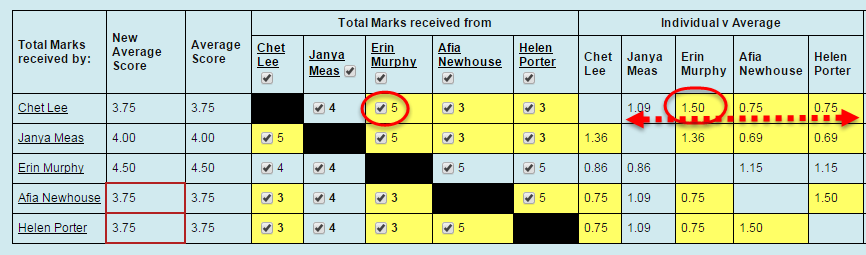## View results

1. Click on the Group Peer Assessment tool link.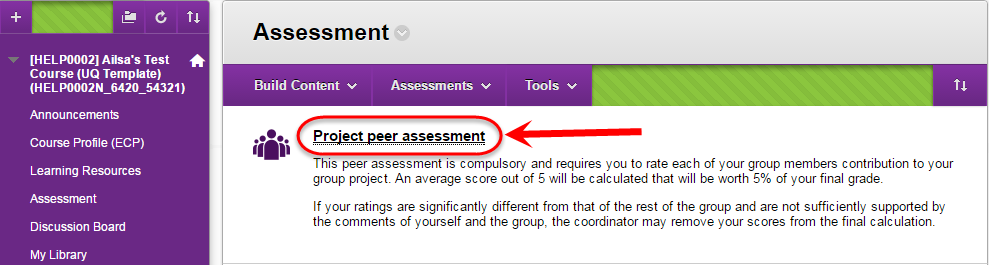1. Select the Scores tab.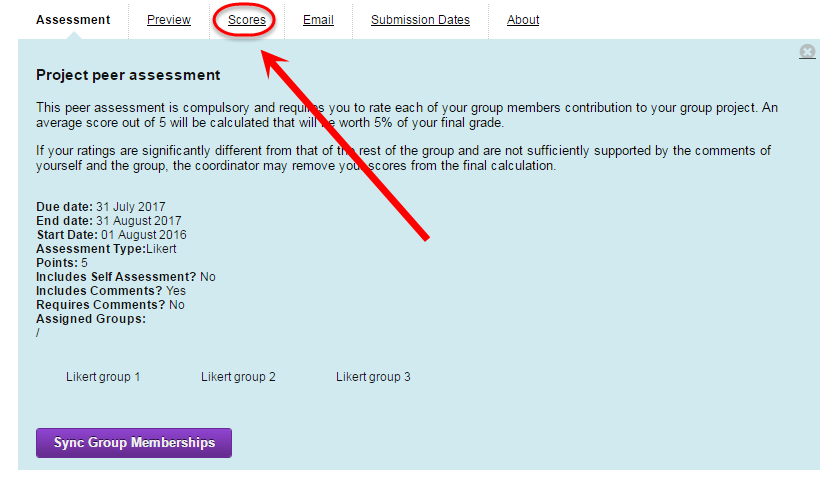## Limit

The limit is used to highlight groups where there is disagreement between members with the scores allocated to individuals. The deeper the yellow highlighting the greater the disagreement.

The default limit is 15% i.e. groups with Ratios of more than 1.15 and less than 0.85. (Refer below for how Ratios are calculated)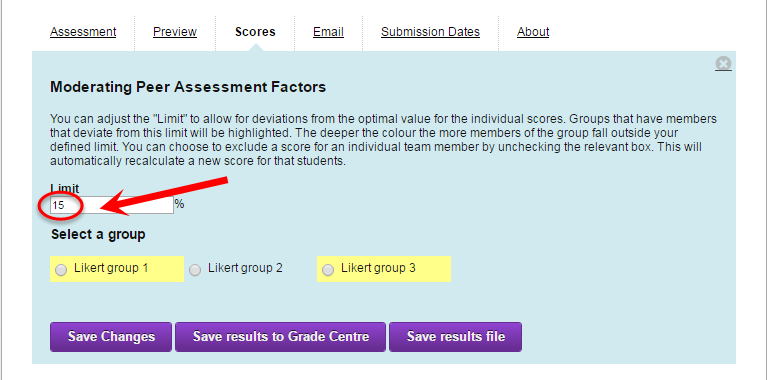### Example

With the Likert option used with a small scale (i.e. 5 points) most scores may be highlighted. In this example the Limit was changed to 30% to highlight the main disagreement in scores.

In the selected group there is disagreement with

1.    The score assigned to Chet from Erin (5), to Afia by Helen (5) and Helen by Afia (5) were highlighted as they were higher than all the other scores assigned to them (3 or 4).

2.    Jana also as a wider range of scores assigned to her 3 or 5.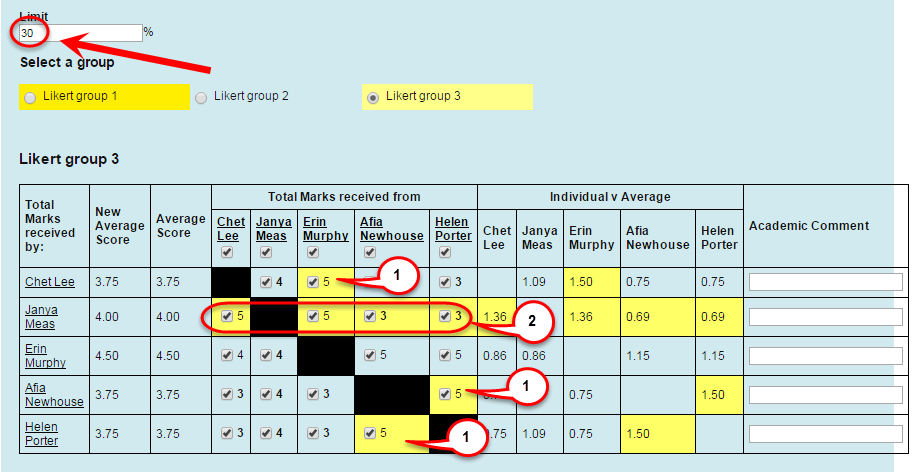## View Total Marks

1. The total marks individual students receive from other group member are shown in the Total Marks section.

### Example

In the example, group members had to rate other group members using a 5 point likert scale for one criteria.

Afia Newhouse in the 4th row received a total mark of 4 from Janya Meas in the 2nd column.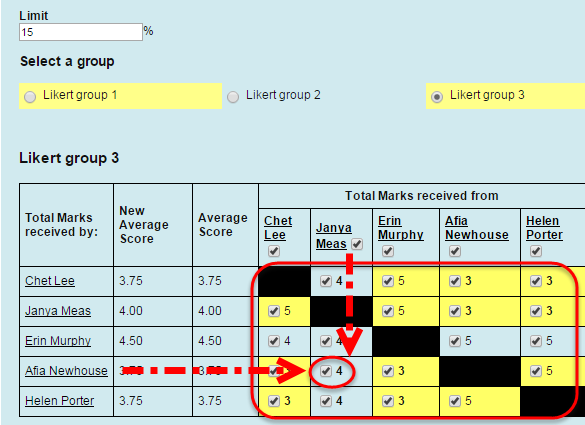1. To view all the marks and feedback an individual group member received click on their name in the appropriate row.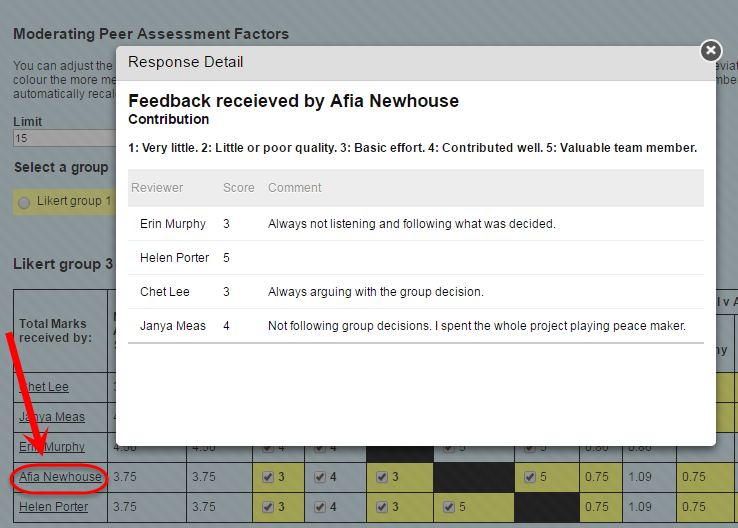1. To view all the marks and feedback an individual group member gave to other group members, select their name in the appropriate column.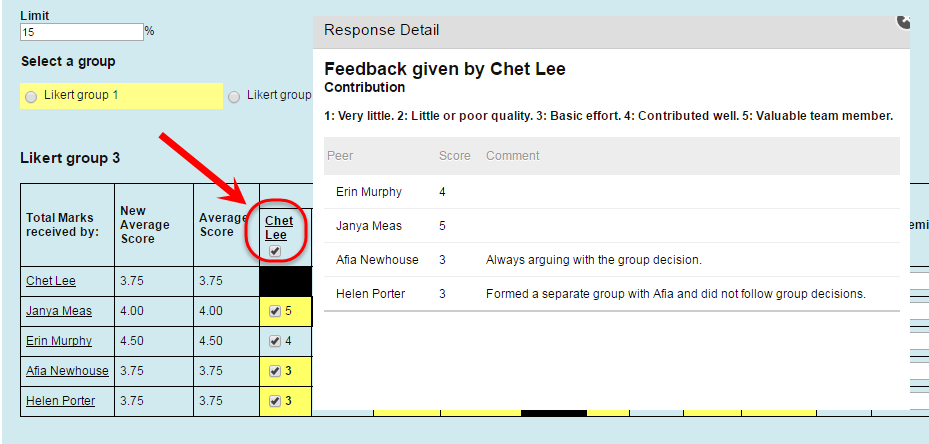## Moderate results

All results should be moderated before being used for summative assessment.

Results could be checked by:

• Checking that the comments justify the scores given.
• Checking if there are common themes with comments from different group members.
• Understanding of feedback from the group’s tutor.
• Meeting with the group or individual group member to discuss the contribution of different group members to the group assignment.

Scores that are not justified can be removed and the Average Score will be recalculated.

Refer below for how Averages and Ratios are calculated.

Note: It is recommended during the moderation process that all student results are checked.

Tip: Use yellow highlights (based on the Limit) to identify groups where there is disagreement between members with the scores allocated to individuals. You can increase the limit to focus on groups with the most disagreement.

Note: It is important that you can justify Likert scores if they are contested.

### Remove all scores assigned by a student

• Uncheck the checkbox next to the student name to remove all the scores they assigned to group members.
• The Average Score will be recalculated and displayed in the New Average column.
• Select the Save Changes button.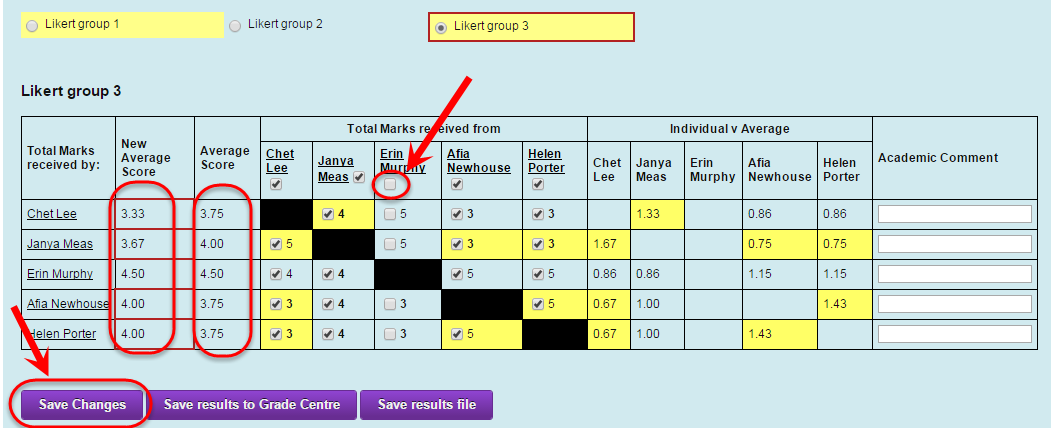### Remove individual scores

• Uncheck the checkbox next to the required score/s.
• The Average Score will be recalculated and displayed in the New Average column.
• Select the Save Changes button.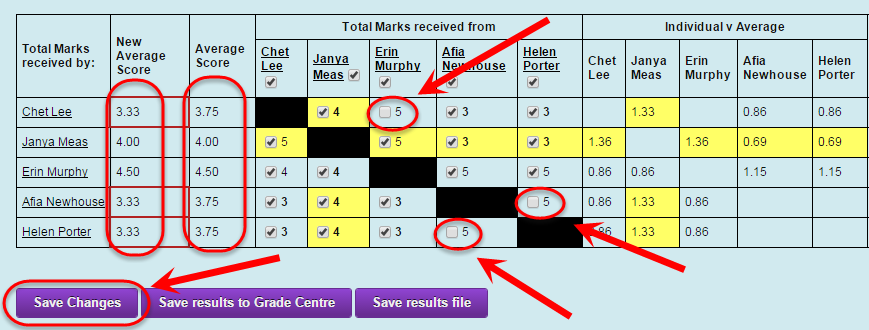## Average Score

The average is calculated to represent an individual student’s contribution to a group assignment. It is the average of all scores awarded from all group members for all criteria. i.e. If the Likert scale is 7, the Average will be out of 7.

### Example Average Score calculation

In the below example group members rate the other group members on one criteria out of 5.

Janya’s Average Score = (5 + 5 + 3 + 3) / 4 = 4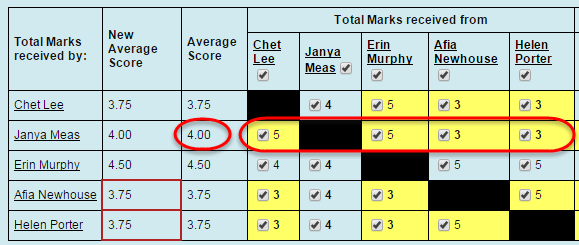## Individual v Average ratio

All individual scores assigned to a student are also compared to those assigned by the rest of the group.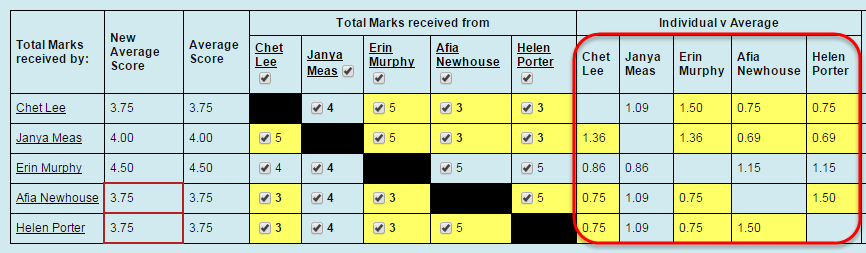### Ratio calculation (Example using Erin giving marks to Chet)

1.Total score assigned from Student A to Student B.

2.Total scores of (other students besides Student A allocated to Student B) / (number of other group members).

3.Divide result from Step 1 by Step 2.

### Example Ratio calculation

The score received by Chet Lee (5) from Erin Murphy does not agree with scores assigned to her by other group members. i.e. Individual v Average ratio of 1.50

### Ratio calculation

= Score from Erin divided by the average score from Janya, Alfia and Helen

= 5 divided by (4 + 3 + 3) / 3

= 5 divided by 3.33

1.50